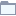# Fuzzy Rules

This category contains 3 nodes.

##### Fuzzy Rule Learner

Learns a Fuzzy Rule Model on labeled numeric data.

##### Fuzzy Rule PredictorStreamable

Applies a Fuzzy Rule Model to numeric data and outputs a prediction for each test instance.

##### Fuzzy Rule PredictorDeprecated

Applies a Fuzzy Rule Model to numeric data and outputs a prediction for each test instance.